When you are calculating numbers that need the same calculation steps repeatedly, you may use the Google Sheets ARRAYFORMULA to simplify your work!

## What is the Google Sheets ARRAYFORMULA?

Google Sheets ARRAYFORMULA is the simplified one time formula, replacing the repeated formula. For an example, if you have a calculation A＊B＋A＊C＋A＊D＋A＊E, by using  ARRAYFORMULA in Google Sheets, you can simplify it to A(B＋C＋D＋E). This will help you to save time and ease your work.

## How to use the Google Sheets ARRAYFORMULA?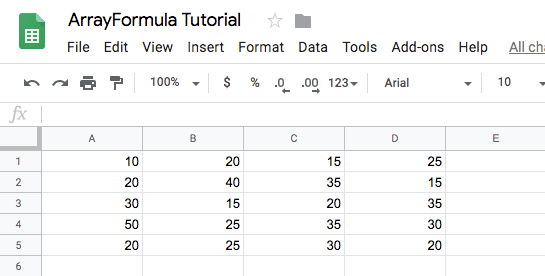From the example above, it is a 4X5 cell table. If you want to calculate the value of each number in the table by 100 times, just type =ARRAYFORMULA(100*A1:D5) to get the answer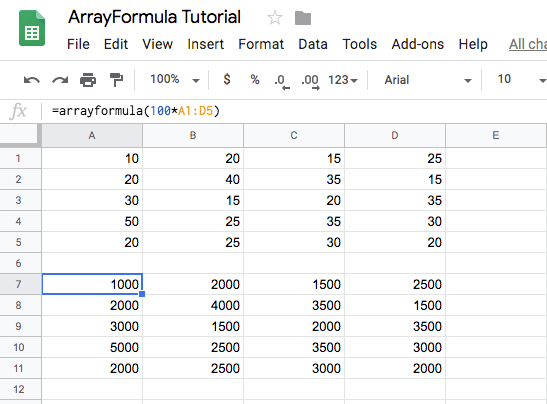You can find that multiple values are shown instead of a single value, and the number of results is equal to the original data.

If you want to know the SUM of these results, and do not want to know the individual results. You can slightly adjust the formula as below:

“=SUM(ARRAYFORMULA(100*A1:D5))”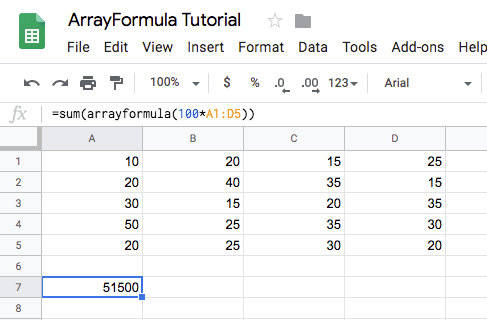## Google Sheets ARRAYFORMULA Practical Use

Let me show you the practical use of ArrayFormula in Google Sheets.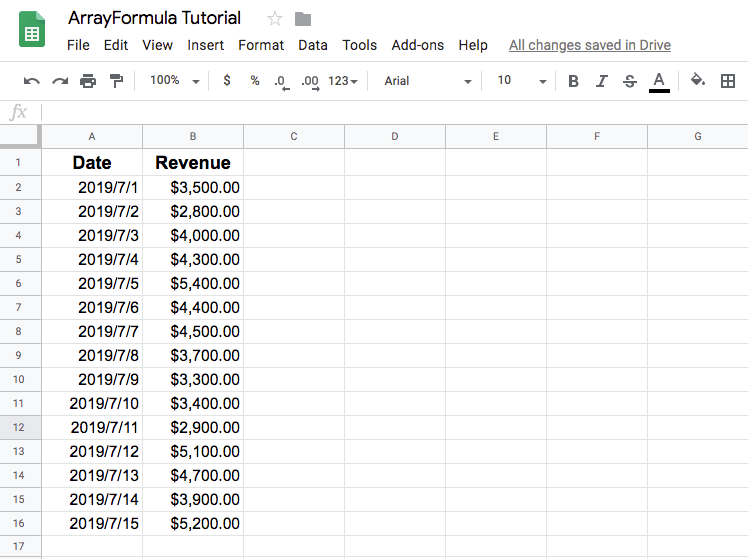Let’s say you’re the owner of a store and you want to figure out how the revenue is generated on different phases. You may curious about how the revenue will be higher at the beginning and lower at the end each month? Therefore, when you want to do this kind of analysis, you can do it through ARRAYFORMULA.

As previously when you want to calculate the average revenue for a certain month, you may use =averageif(DAY(A:A),D2,B:B) formula. However, it will show the evaluation of your formula is error.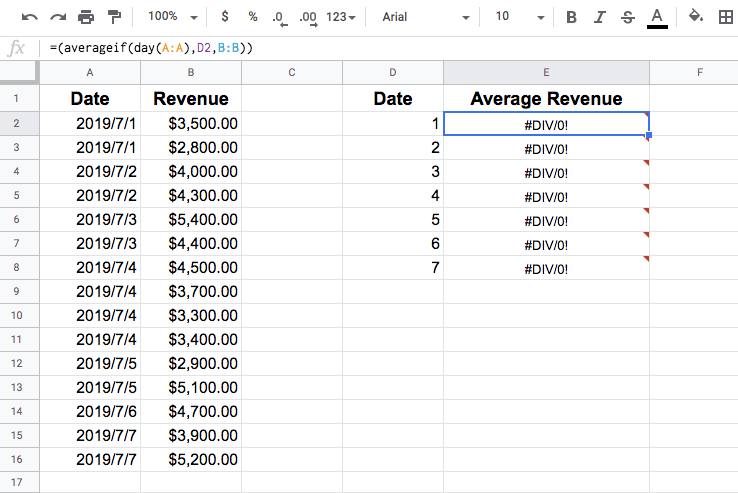This error is because the”day” only be used to calculate the data”in a specific date or cell”. There is no way to calculate all the data in the range at one time. Therefore, the conditional judgment result of the above formula is not valid, and the divisor is zero

However, if we know how to use”ArrayFormula”in Google Sheets, we can do it 🙂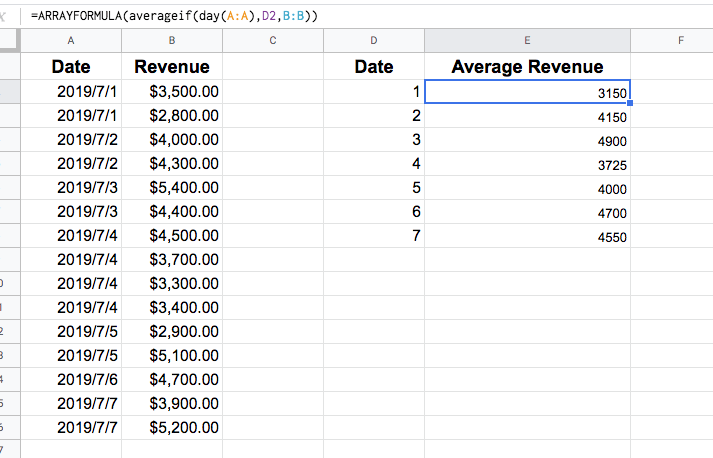This is the best time to use the Google Sheets ARRAYFORMULA. Sometimes it is impossible to calculate all the data in a range at one time. Therefore, you can use the ARRAYFORMULA in Google Sheets to achieve the desired calculation.